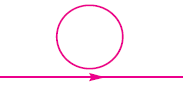A current carrying wire is placed below a coil in its plane, with current flowing as shown. If the current increases.

# A current carrying wire is placed below a coil in its plane, with current flowing as shown. If the current increases.1. A

No current will be induced in the coil

2. B

An anti clockwise current will be induced in the coil

3. C

A clockwise current will be induced in the coil

4. D

The current induced in the coil will be first anti clockwise and then clockwise

Register to Get Free Mock Test and Study Material

+91

Verify OTP Code (required)

### Solution:

As the current in the wire is constantly increasing, flux through the loop is also increasing. Field lines are directed out of the   plane of the paper.

By lenz's law, current flows in the loop so as to oppose the effect. Hence, it flows in clockwise direction as shown in the figure.Register to Get Free Mock Test and Study Material

+91

Verify OTP Code (required)